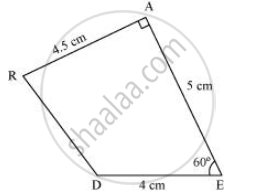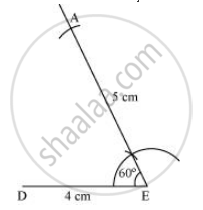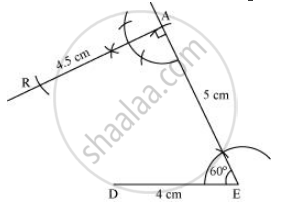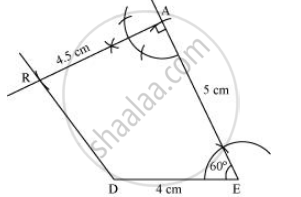Share

# Construct the Following Quadrilaterals, Quadrilateral Dear De = 4 Cm Ea = 5 Cm Ar = 4.5 Cm ∠E = 60° ∠A = 90° - CBSE Class 8 - Mathematics

ConceptConstructing a Quadrilateral When Three Sides and Two Included Angles Are Given

DE = 4 cm

EA = 5 cm

AR = 4.5 cm

∠E = 60°

∠A = 90°

#### Solution

1) A rough sketch of this quadrilateral can be drawn as follows.2) Draw a line segment DE of 4 cm and an angle of 60º at point E. As vertex A is 5 cm away from vertex E, cut a line segment EA of 5 cm from this ray.3) Again draw an angle of 90º at point A. As vertex R is 4.5 cm away  from vertex A, cut a line segment RA of 4.5 cm from this ray.4) Join D to R.